# JavaScript list (array) comprehension explainedself.__wrap_n=self.__wrap_n||(self.CSS&&CSS.supports("text-wrap","balance")?1:2);self.__wrap_b=(e,r,t)=>{t=t||document.querySelector(`[data-br="\${e}"]`);let n=t.parentElement,a=s=>t.style.maxWidth=s+"px";t.style.maxWidth="";let o,i=n.clientWidth,l=n.clientHeight,c=i/2-.25,f=i+.5;if(i){for(a(c),c=Math.max(t.scrollWidth,c);c+1<f;)o=Math.round((c+f)/2),a(o),n.clientHeight===l?f=o:c=o;a(f*r+i*(1-r))}t.__wrap_o||typeof ResizeObserver<"u"&&(t.__wrap_o=new ResizeObserver(()=>{self.__wrap_b(0,+t.dataset.brr,t)})).observe(n)};self.__wrap_n!=1&&self.__wrap_b(":Rj0pm:",1)

November 6, 2023

JavaScript arrays are versatile data structures that let you store multiple values in a single variable. List comprehension is a concise way to create and manipulate arrays based on existing arrays or iterables. In languages like Python, list comprehension is a specific feature. In JavaScript, however, you do this with methods like `.map()`, `.filter()`, and array destructuring.

## Understanding arrays in JavaScript

An array in JavaScript is a global object that allows you to store a list of items. You can create an array using square brackets `[]` and access its elements through their indexes. Arrays in JavaScript are dynamic, and you can perform a variety of operations on them, like adding, removing, or modifying elements.

```let fruits = ["apple", "banana", "cherry"];
console.log(fruits); // Outputs: apple```

## Create a new array from an existing one

You can create a new array from an existing one by applying a function to each element of the array. The `.map()` method is commonly used for this purpose.

```let numbers = [1, 2, 3, 4];
let squares = numbers.map(x => x * x);
console.log(squares); // Outputs: [1, 4, 9, 16]```

## Filter an array based on a condition

To filter an array based on a condition, use the `.filter()` method. This method creates a new array with all elements that pass the test implemented by the provided function.

```let numbers = [1, 2, 3, 4];
let evenNumbers = numbers.filter(x => x % 2 === 0);
console.log(evenNumbers); // Outputs: [2, 4]```

## Combine map and filter

For more complex list comprehensions, you can chain `.map()` and `.filter()` methods to first filter an array, then apply a function to the filtered results.

```let numbers = [1, 2, 3, 4];
let doubledEvens = numbers.filter(x => x % 2 === 0).map(x => x * 2);
console.log(doubledEvens); // Outputs: [4, 8]```

## Use array destructuring for comprehension

Array destructuring is a JavaScript expression that allows you to unpack values from arrays, or properties from objects, into distinct variables. You can use destructuring in combination with `.map()` to perform more complex transformations.

```let pairs = [[1, 'one'], [2, 'two'], [3, 'three']];
let numbers = pairs.map(([number, name]) => number);
console.log(numbers); // Outputs: [1, 2, 3]```

## Reduce an array to a single value

The `.reduce()` method is used to apply a function against an accumulator and each element in the array to reduce it to a single value.

```let numbers = [1, 2, 3, 4];
let sum = numbers.reduce((accumulator, currentValue) => accumulator + currentValue, 0);
console.log(sum); // Outputs: 10```

The spread syntax `...` allows an iterable such as an array to be expanded in places where zero or more arguments are expected. It can be used to concatenate arrays or to insert an array into another array.

```let numbers = [1, 2, 3];
let moreNumbers = [4, 5, 6];
let combined = [...numbers, ...moreNumbers];
console.log(combined); // Outputs: [1, 2, 3, 4, 5, 6]```

There are a couple other methods like `.some()`, `.every()`, `.find()`, and `.flatMap()` that are worth thinking about:

• `.some()` checks if at least one element in the array passes the test implemented by the provided function.
• `.every()` checks if all elements in the array pass the test implemented by the provided function.
• `.find()` returns the value of the first element in the array that satisfies the provided testing function.
• `.flatMap()` first maps each element using a mapping function, then flattens the result into a new array.
```// Example of .flatMap()
let nestedArrays = [, [2, 3], ];
let flattened = nestedArrays.flatMap(x => x);
console.log(flattened); // Outputs: [1, 2, 3, 4]```

## Custom iterator functions

For advanced array operations, consider creating custom iterator functions:

```// Example of a custom iterator function
function* range(start, end) {
for (let i = start; i <= end; i++) {
yield i;
}
}

let rangedArray = [...range(1, 5)];
console.log(rangedArray); // Outputs: [1, 2, 3, 4, 5]```

## Performance

When dealing with large datasets or performance-critical applications, consider the efficiency of your array operations. Methods like `.map()` and `.filter()` create new arrays, which could impact memory usage. Loop fusion, where you combine operations to minimize iterations over the data, can improve performance.

Here's how you can use `.reduce()` for array flattening:

```// Example of using .reduce() to flatten an array
let nestedArrays = [, [2, 3], ];
let flattened = nestedArrays.reduce((acc, val) => acc.concat(val), []);
console.log(flattened); // Outputs: [1, 2, 3, 4]```

## Asynchronous operations

Combine array methods with Promises and async/await for handling asynchronous operations elegantly:

```// Example of using async/await with .map()
async function getPosts(ids) {
let posts = await Promise.all(ids.map(id => fetchPostById(id)));
return posts;
}```

# Ship faster, worry less with Basedash

You're busy enough with product work to be weighed down building, maintaining, scoping and developing internal apps and admin panels. Forget all of that, and give your team the admin panel that you don't have to build. Launch in less time than it takes to run a standup.

You're busy enough with product work to be weighed down building, maintaining, scoping and developing internal apps and admin panels. Forget all of that, and give your team the admin panel that you don't have to build. Launch in less time than it takes to run a standup.

You're busy enough with product work to be weighed down building, maintaining, scoping and developing internal apps and admin panels. Forget all of that, and give your team the admin panel that you don't have to build. Launch in less time than it takes to run a standup.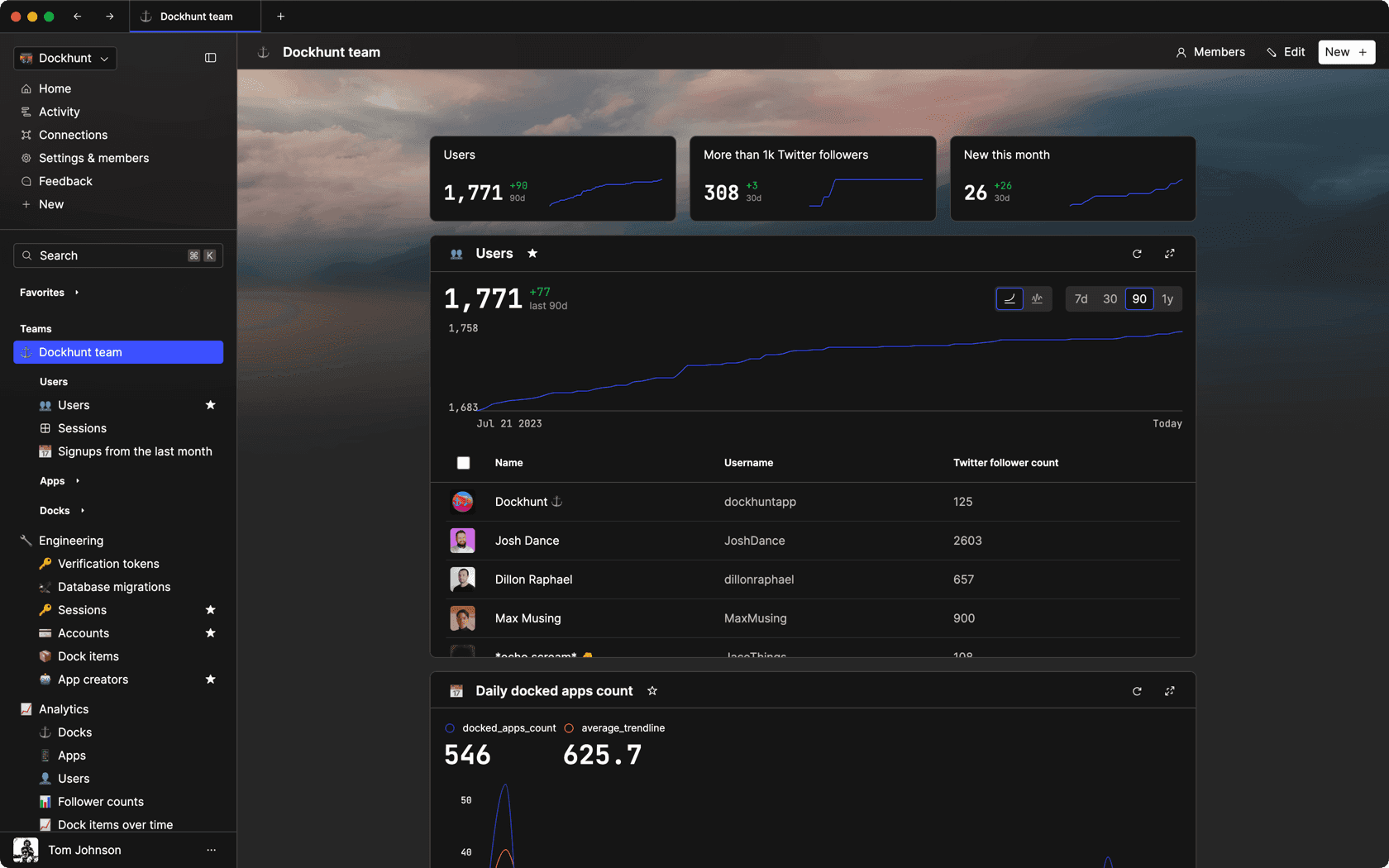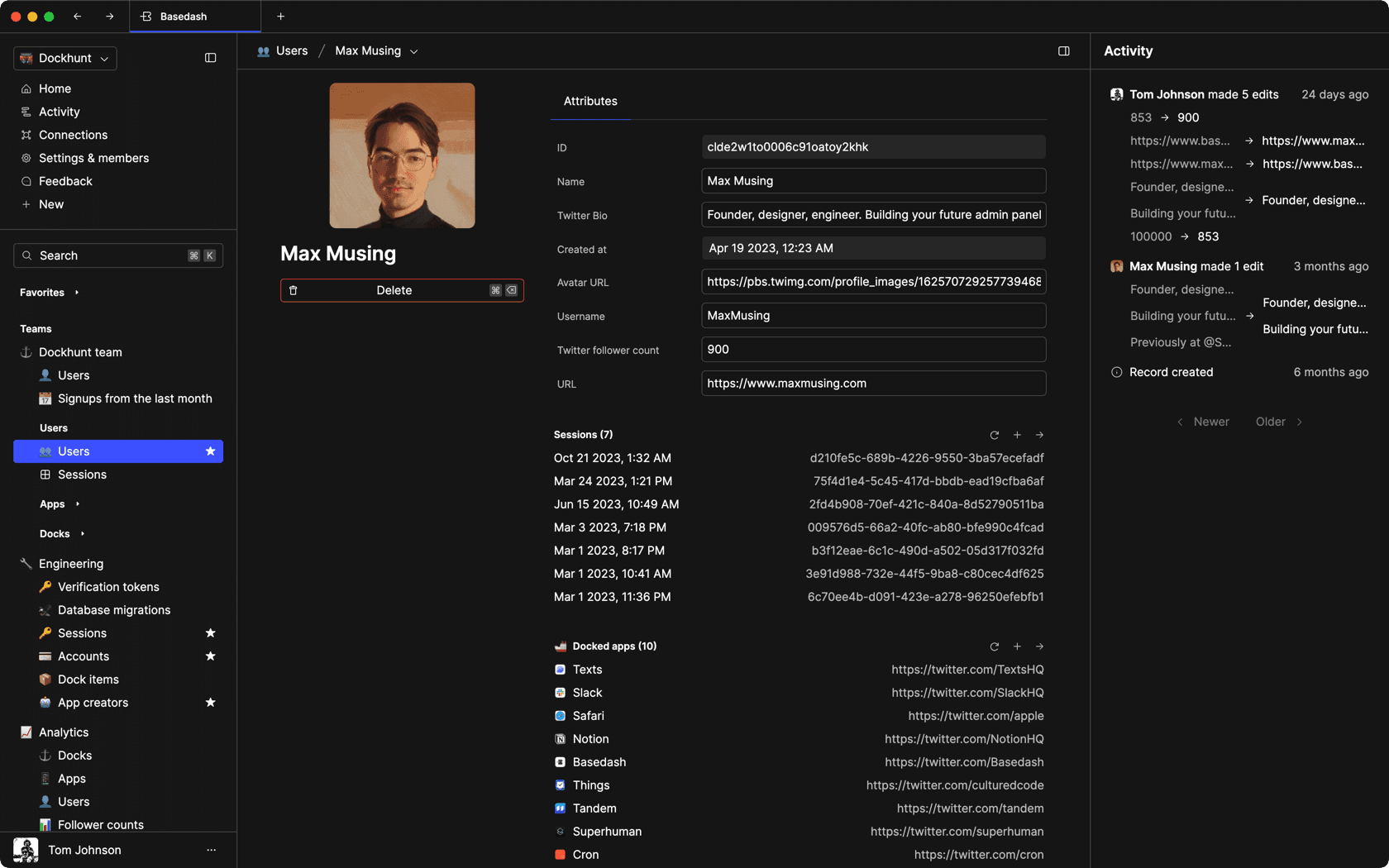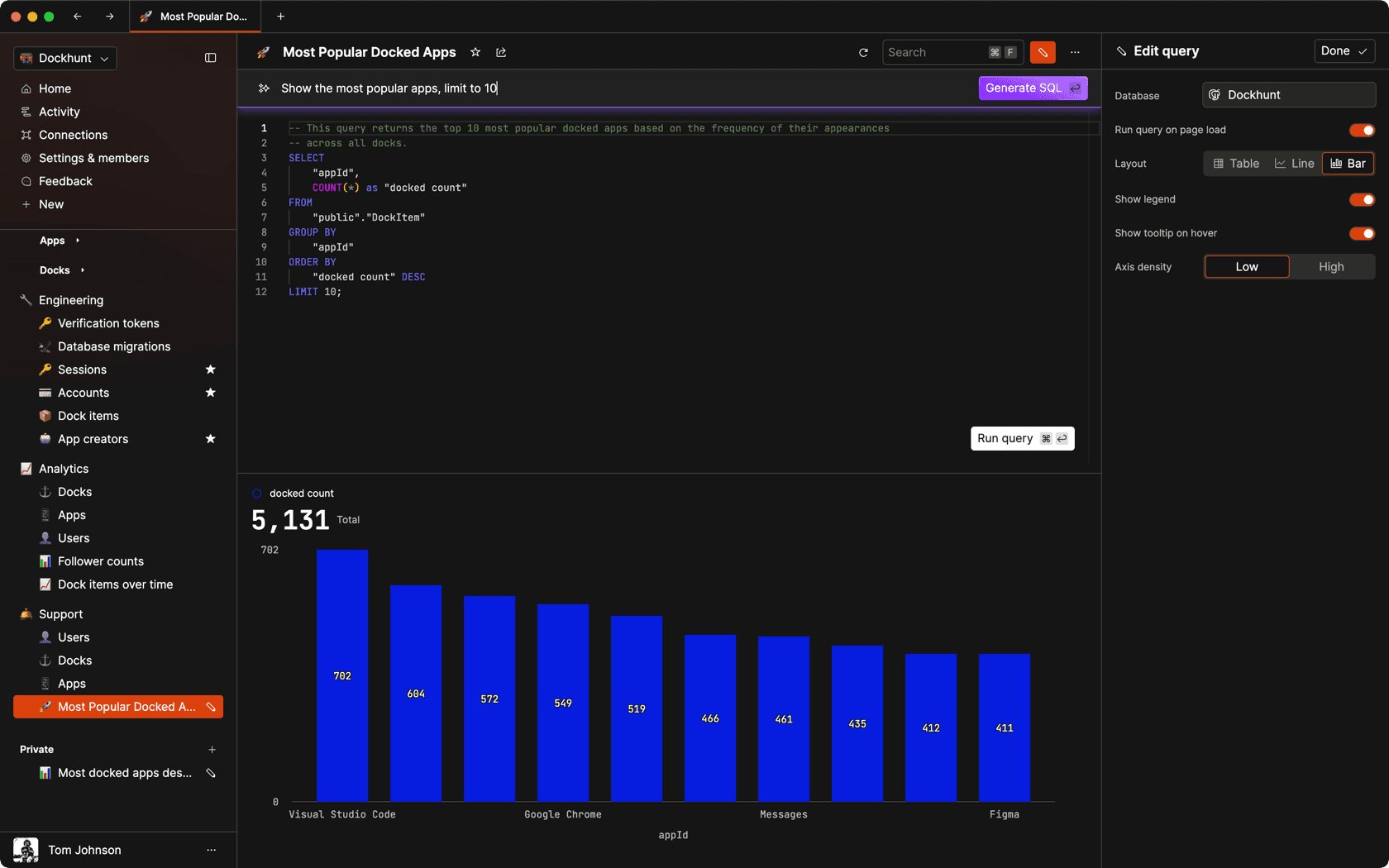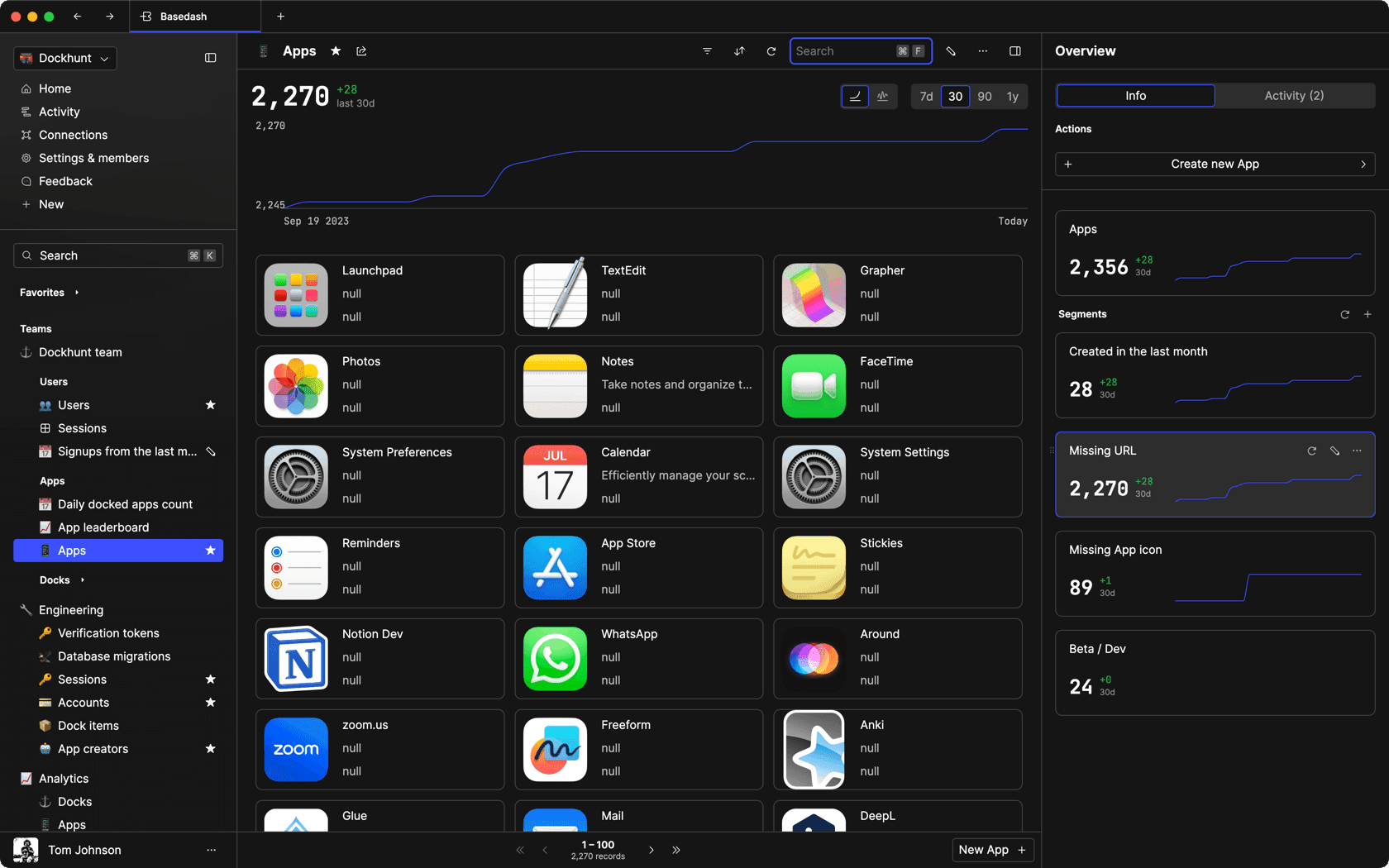### Dashboards and charts

Effortlessly make charts and create a space for your team to work together towards shared goals and metrics.

### SQL composer with AI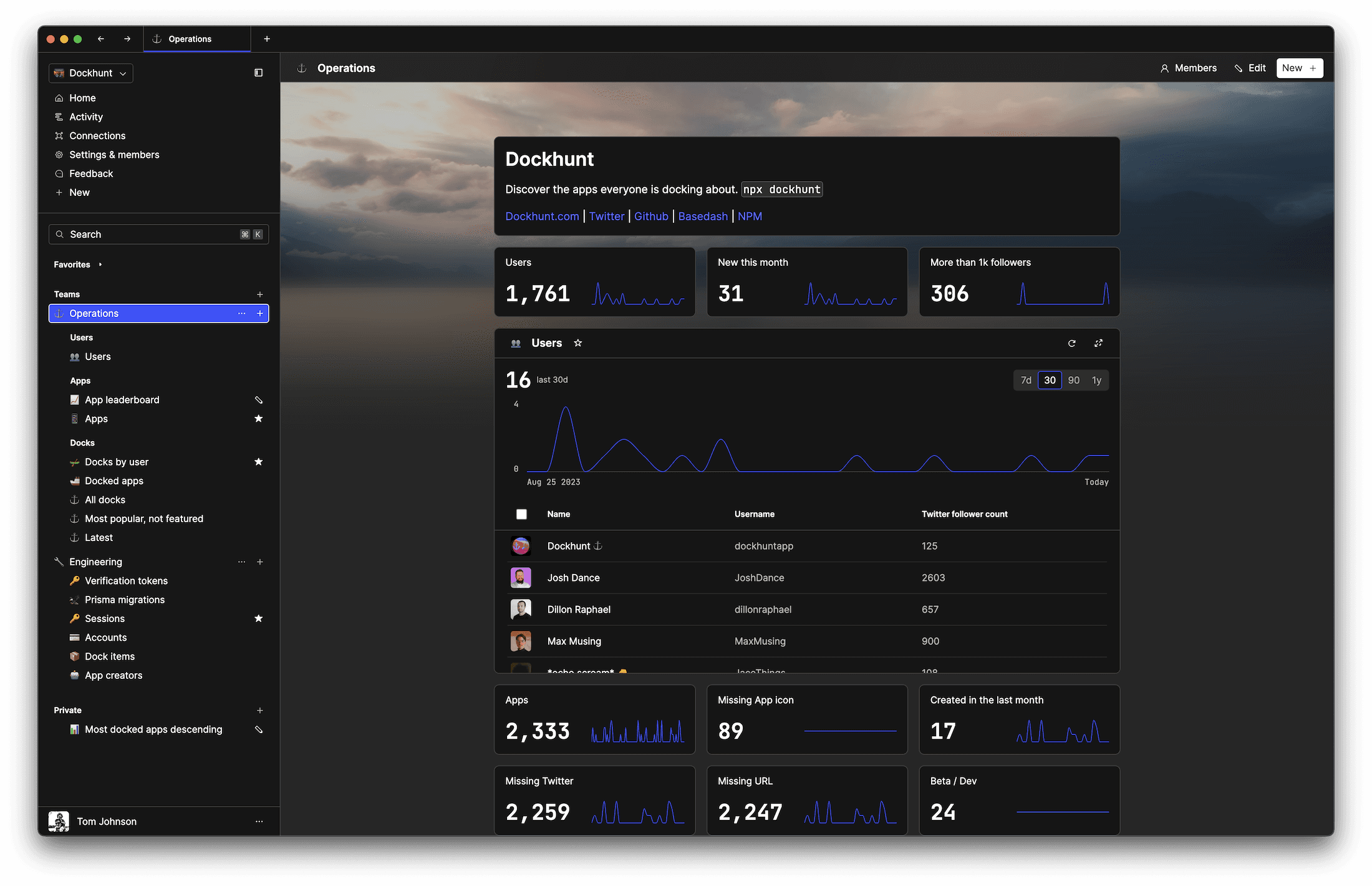SOC II
Type 2 Certified

### Features

Proudly carbon-neutral.SOC II
Type 2 Certified

### Features

Proudly carbon-neutral.SOC II
Type 2 Certified